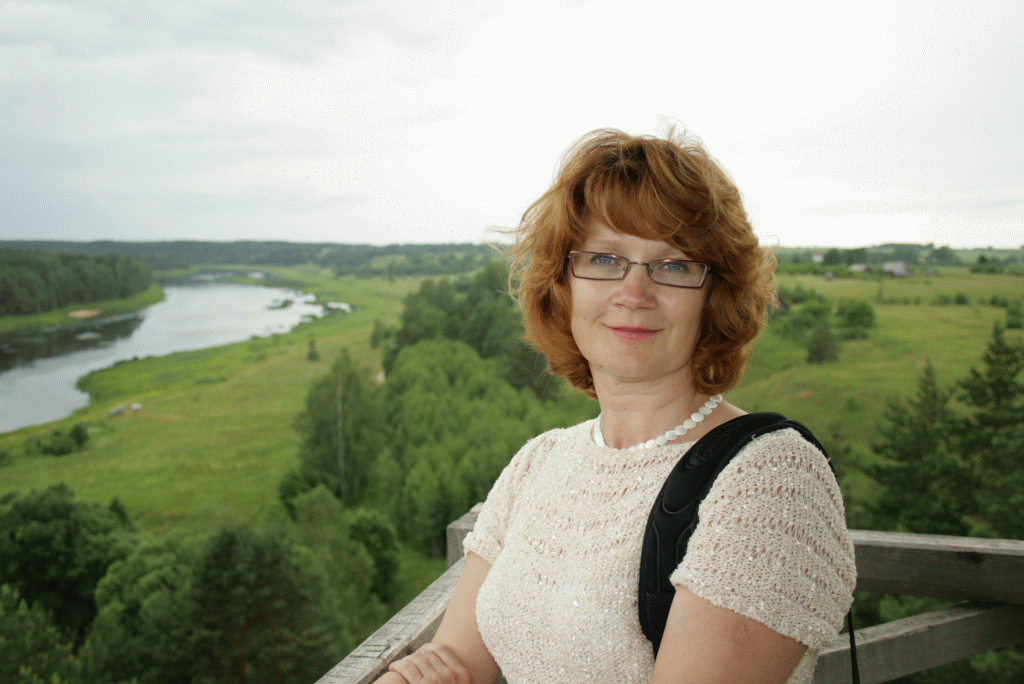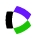Inara YermachenkoLeading researcher, associate professor, Dr.math. Mathematical Research Center Department of Technology Institute of Life Sciences and Technology Daugavpils University Parades street 1 LV-5400, Daugavpils, Latvia inara.jermacenko@du.lvResearch interests Nonlinear boundary value problems for ordinary differential equations, didactics of modern elementary mathematics, mathematical education Education Dr. math, Daugavpils University, Latvia, 2007, Ph.D. thesis "Quasilinearization and types of solution to nonlinear boundary value problems" Advisor: prof. F. Sadyrbaev. Teaching Academic bachelor study programme "Mathematics": ordinary differential equations, equations of mathematical physics, numerical analysis, real functions Academic master study programme "Mathematics": boundary value problems for ordinary differential equations, partial differential equations, calculus of variations, methods of modern elementary mathematics Recent publications A. Gritsans and I. Yermachenko, On the maximum number of period annuli for second order conservative equations, Vol. 26, No. 4,  2021, pp. 612-630. , A. Kolyshkin, D. Ogorelova, , I. Samuilik, and I. Yermachenko, Solutions of nonlinear boundary value problem with applications to biomass thermal conversion, Proceedings of 20th International Scientific Conference "Engineering for rural development" (May 26-28, Jelgava, Latvia), 2021, pp. 837-842. F. Sadyrbaev and I. Yermachenko. Dirichlet boundary value problem for a system of n second order asymptotically asymmetric differential equations, Electron. J. Differential Equations, Vol. 2018 (2018), No. 35, pp. 1-16. F. Sadyrbaev and I. Yermachenko. Dirichlet boundary value problem for the second order asymptotically linear system. International Journal of Differential Equations, V. 2016 (2016), Article ID 5676217, 12 pages http://dx.doi.org/10.1155/2016/5676217. I. Yermachenko and . On a problem for a system of two the second order differential equations via the theory of vector fields. Nonlinear Analysis. Modelling and Control, Vol. 20, No. 2, 2015, 175-189. M. Dobkevich, , N. Sveikate and I. Yermachenko. On types of solutions of the second order nonlinear boundary value problems. Abstract and Applied Analysis, V. 2014 (2014), Article ID 594931, 9 pages, http://dx.doi.org/10.1155/2014/594931. Hindawi I. Yermachenko and . Quasilinearization and multiple solutions of the second order nonlinear boundary value problem, Journal of Control Engineering and Technology, Vol. 4, N. 1, 2014, 1-8. EBSCO pdf I. Yermachenko and . Quasilinearization technique for Φ-Laplacian type equations. International Journal of Mathematics and Mathematical Sciences, V. 2012 (2012), Article ID 975760, 11 pages doi:10.1155/2012/975760. Hindawi I. Yermachenko. Maximum principle and the fourth order boundary value problem. Mathematical Modelling and Analysis, V. 16, N. 1,  2011, 143-152. I. Yermachenko. Two-point boundary value problems at resonance. Mathematical Modelling and Analysis, V. 14, N. 2,  2009, 247-257. and I. Yermachenko. Multiple solutions of nonlinear boundary value problems for two-dimensional differential systems. Dynamical Systems and Differential Equations.   Proc. of  the 7th AIMS International Conference (Arlington, TX, USA, 2008), DCDS Supplement 2009, 659 - 668. American Institute of Mathematical Sciences and I. Yermachenko. Multiple solutions of two-point nonlinear boundary value problems. Nonlinear Analysis: TMA, V. 71, N. 12, 2009, e176 – e185, Proc. WCNA 2008, Orlando FL, USA, 2008. Elsevier I. Yermachenko. Multiple solutions of the BVP for two-dimensional system by extracting linear parts and quasilinearization. Mathematical Modelling and Analysis, V. 13, N. 2,  2008, 303-312. I. Yermachenko. On solvability of the BVPs for the fourth order Emden-Fowler equation. Mathematical Modelling and Analysis, V. 12, N. 2,  2007, 267-276. I. Yermachenko. Multiple solutions of nonlinear boundary value problems by the quasilinearization process. Proceedings of Equadiff 11, International Conference on Differential Equations. Czecho-Slovak series, Bratislava, July 25-29, 2005. [Part 2] Minisymposia and contributed talks. Comenius University Press, Bratislava, 2007, pp. 577-587. I. Yermachenko and . Types of solutions and multiplicity results for two-point nonlinear boundary value problems. Proceedings of AIMS' Sixth International Conference on Dyn. Systems, Diff. Equations and Applications University of Poitiers, Poitiers, France, June 25 - 28, 2006, Discrete and Continuous Dynamical Systems -  Supplement 2007, American Institute of Mathematical Sciences, 1061-1069. American Institute of Mathematical Sciences I. Yermachenko. Multiple solutions of the fourth order Emden-Fowler equation. Mathematical Modelling and Analysis, V. 11, N. 3,  2006, 347-356. I. Yermachenko and FF. Sadyrbaev. Types of solutions and multiplicity results for two-point non­linear boundary value problems. Nonlinear Analysis: TMA, V. 63, N. 5-7, 2005, e1725 – e1735. Elsevier I. Yermachenko and . Quasilinearization and multiple solutions of the Emden-Fowler type equation.  Mathematical Modelling Analysis, Vol. 10, No. 1, 2005, 41-50. Activities Fundamental and applied research project of the Latvian Council of Science "Analysis of complex dynamical systems in fluid mechanics and heat transfer", Researcher,  lzp-2021/1-0076. Member of the Latvian Mathematical Society Member of Organizing Committee to School of Young mathematicians, Daugavpils University European Social Fund Project “Science and Mathematics” (2008/0002/1DP/1.2.1.2.1/08/IPIA/VIAA/001), implementer and expert